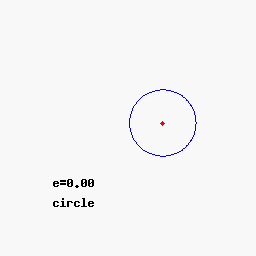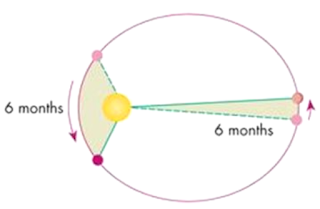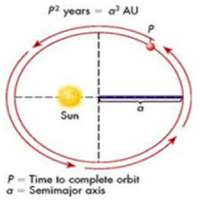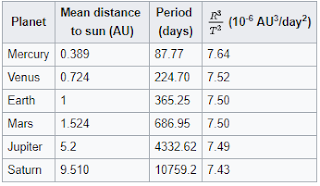### Section 4.2 - Kepler's Laws of Planetary Motion

#### Conic Sections

In a throwback to my Algebra II class in high school, conic sections are extremely important for orbital mechanics. As a refresher, the four conic sections are the figures that can be formed by the intersection of a plane and a right circular cone

Technically speaking, conic sections are defined as the set of all points whose distance to a fixed point (the focus) is a constant multiple (the eccentricity) of the distance to a fixed line (the directrix)A circle is a special type of ellipse with e = 0. Ellipse: 0 < e < 1 Parabola: e = 1 Hyperbola: e > 1

Don't worry, for our purposes we only need to know a few characteristics of conic sections, but if you want more detail behind the math, I recommend this website.Visualization of the conic sections as eccentricity increases

#### Kepler's Laws of Planetary Motion

Between 1609 and 1618, German astronomer Johannes Kepler accurately described the motions of the solar system through his three laws, and it didn't take long to generalize them to all orbits

• Law 1: The orbit of a planet is an ellipse with the Sun at one of the two fociAn ellipse is defined as all the points in a plane such that the sum of the distances to two other points, the two foci, is the same. See this image

• Law 2: A line segment joining a planet and the Sun sweeps out equal areas over equal timesWhen the planet is close to the Sun it speeds up, but when it's far a way it slows down. The net effect is that the shaded areas that get swept across over a certain time period (6 months in this case) are always equal

• Law 3: The square of the orbital period is proportional to the cube of the semi-major axis of the orbit (the semi-major axis is just the line from the center of the ellipse to one of the wider ends)In mathematical terms, $P^2 \propto a^3$Kepler's data and calculations in 1618. Notice how $P^2$ and $a^3$ all come out to the same ratio for each of planets "I first believed I was dreaming... but it is absolutely certain that the ratio which exists between the period times of any two planets is precisely the ratio of the 3/2th power of the mean distance." - Johannes Kepler, Harmonies of the World (1619)